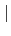## Staggered Problem

 StaggeredProblem (nsteps #(in) deltaT #(rn))timeDefinedByProb #(in) prob1 #(s) prob2 #(s) [stepMultiplier #(rn)]

Represent so-called staggered analysis. This can be described as an sequence of sub-problems, where the result of some sub-problem in the sequence can depend on results of previous sub-problems in sequence. Typical example is heat transfer analysis followed by mechanical analysis taking into account the temperature field generated by the heat transfer analysis. Similar analysis can be done when coupling moisture transport with concrete drying strain.

The actual implementation supports only sequence of two sub-problems. The sub-problems are described using sub-problem input files. The syntax of sub-problem input file is the same as for standalone problem. The only addition is that sub-problems should export their solution fields so that they became available for subsequent sub-problems. See the Section 3.2.1.

The subproblem input files are described using prob1 and prob2 parameters, which are strings containing a path to sub-problem input files, the prob1 contains input file path of the first sub-problem, which runs first for each solution step, the prob2 contains input file path of the second sub-problem.

There are two options how to control a time step sequence. The first approach uses timeDefinedByProb which uses time sequence from the corresponding subproblem. The subproblem may specify arbitrary loading steps and allows high flexibility. The second approach uses the staggered problem to take control over time. Therefore any sub-problem time-stepping parameters are ignored (even if they are required by sub-problem input syntax) and only staggered-problem parameters are relevant. deltaT is than a time step length used for integration, nsteps parameter specifies number of time steps to be solved. stepMultiplier multiplies all times with a given constant. Default is 1.

Note: This problem type is included in transport module and it can be used only when this module is configured. Note: All material models derived from StructuralMaterial base will take into account the external registered temperature field, if provided.

Borek Patzak
2018-01-02